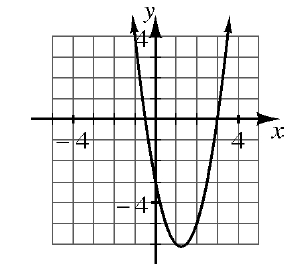### Home > GC > Chapter 3 > Lesson 3.2.3 > Problem3-68

3-68.

On graph paper, graph the parabola $y = 2x^2 - 5x - 3$. 3-68 HW eTool (Desmos).What are the roots ($x$-intercepts) of the parabola?Hint (a):Look at the graph. Where does the parabola cross the $x$-axis?Read the Math Notes box for this lesson. Then solve the equation $2x^2 - 5x - 3 = 0$ algebraically. Did your solutions match your roots from part (a)?Hint (b):Use the Quadratic Formula.Answer (b):$\text{One solution is }x=\frac{-(-5)+\sqrt{(-5)^{2}-4(2)(-3)}}{2(2)},\text{ or }x=3.$

Use the eTool below to graph the parabola.
Click the link at right for the full version of the eTool: GC 3-68 HW eTool